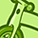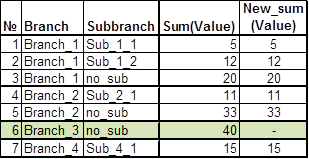Announcements
MAINTENANCE ALERT: Dec. 12th starting 9 AM CET. Community will be read-only. GET DETAILS
cancel
Showing results for
Did you mean:Contributor III

## Set analisys: sum with specific condition

Good day!

I got some data:I need to make expression for New_sum(Value) with condition:

- if there is only Subbranch "no_sub" in Branch, then we don't sum value. If there are other Subbranches, then we sum all of them.

1 Solution

Accepted SolutionsMVP

May be like this:

Sum({<Branch = {"=Count(DISTINCT Subbranch) = 1 and Subbranch = 'no_sub' "}>}Value)

=Sum({<Branch = {"=Not(Count(DISTINCT Subbranch) = 1 and Subbranch = 'no_sub')"}>}Value)

3 RepliesMVP

May be like this:

Sum({<Branch = {"=Count(DISTINCT Subbranch) = 1 and Subbranch = 'no_sub' "}>}Value)

=Sum({<Branch = {"=Not(Count(DISTINCT Subbranch) = 1 and Subbranch = 'no_sub')"}>}Value)MVP

Or this:

=Sum({<Branch = {"=Count(DISTINCT Subbranch) <> 1 or Subbranch <> 'no_sub' "}>}Value)Contributor III
Author

Sunny, great! thank you!Community Browser